# php 一维数组怎么增加元素

php一维数组增加元素的方法

array_unshift()函数可以在数组的开头插入一个或多个新元素（键值）。

`array_unshift(\$array,\$value1,\$value2...)`

```<?php
\$arr=array(10,12,20);
array_unshift(\$arr,8,"9");
var_dump(\$arr);
?>```

`array_unshift(\$arr,8,9)`可以看出是在\$arr数组的开头插入两个新元素：数值“`8`”和字符串“`9`”，因此输出结果为：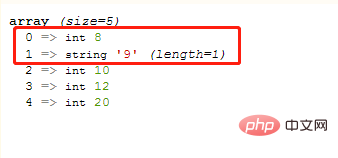array_unshift()函数不会保持原有的数值索引关系，会删除所有的数值键名，并为其重新赋值，即从 0 开始重新计数；但所有的字符串键名保持不变。

```<?php
\$arr=array("a"=>"red","b"=>"green",3=>"pink");
echo "原来的数组：";
var_dump(\$arr);
array_unshift(\$arr,"blue");
echo "在开头插入一个新元素后：";
var_dump(\$arr);
?>```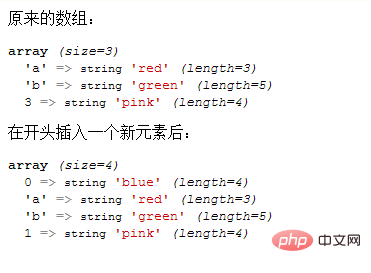array_splice()函数是一个强大的函数，可以用来删除数组元素、替换数组元素，也能用来插入数组元素（只需要将参数\$length的设置为0即可）。

`array_splice(\$array,\$start,\$length,\$value)`

`\$length=0`，那么参数`\$start`就可指定开始插入的位置（下标），参数`\$value`就可指定插入值（如果是多个值就需要设置为数组）。

array_splice()函数既可以在数组开头添加元素，也可在尾部增加元素。

• 而想要在开头添加元素，那么\$start的值需要为0。

```<?php
\$arr1=array(10,12,20);
var_dump(\$arr1);
array_splice(\$arr1,0,0,"1");
var_dump(\$arr1);

\$arr2=array(10,12,20);
array_splice(\$arr2,0,0,array("1",25,"3"));
var_dump(\$arr2);
?>```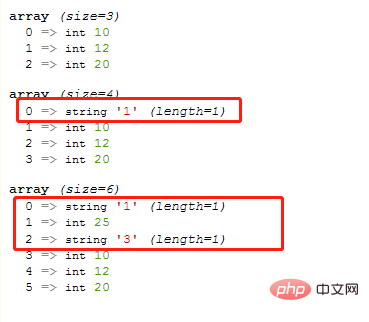• 而当将`\$start`的值设置为“数组长度值”，即`count(\$arr)`即可在数组末尾插入元素。

```<?php
\$arr1=array(10,12,20);
array_splice(\$arr1,count(\$arr1),0,"1");
var_dump(\$arr1);

\$arr2=array(10,12,20);
array_splice(\$arr2,count(\$arr2),0,array("1",25,"3"));
var_dump(\$arr2);
?>```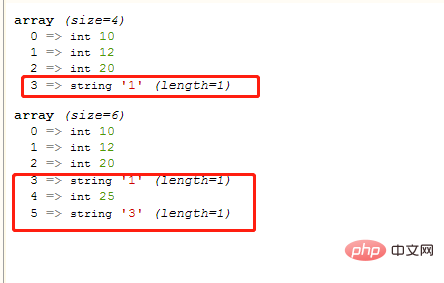array_push()函数可以在数组尾部插入一个或多个元素（键值），语法如下：

`array_push(\$array,\$value1,\$value2...)`

```<?php
\$arr=array(10,12,20);
array_push(\$arr,8,"9",3.14);
var_dump(\$arr);
?>```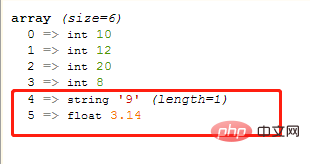array_push()函数插入的元素可以是数组类型

```<?php
\$arr=array(10,12,20);
var_dump(\$arr);
array_push(\$arr,array(1,2,3));
var_dump(\$arr);
?>```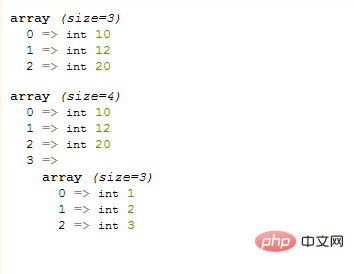`array_pad(\$array,\$size,\$value)`函数可以将某个键值`\$value`插入到数组`\$array`中，从而将数组填补到指定的长度`\$size`。（`\$size`参数可以理解为数组中元素的最终数目，即插入操作后的数组长度）。

• 当\$size参数为正数，则在数组尾部插入元素。

```<?php
\$arr=array(10,12,20);
var_dump(\$result);
?>```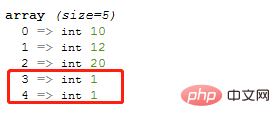```<?php
\$arr=array(10,12,20);
var_dump(\$result);
?>```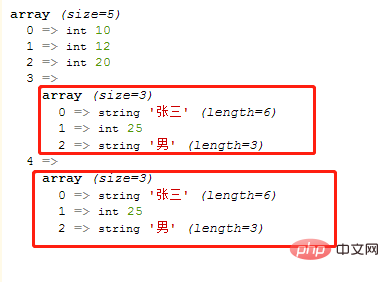`array_merge(array1,array2,array3...)` 函数用于把一个或多个数组合并为一个数组，将`array2,array3...`的值存入`array1`中。

```<?php
\$arr=array(10,12,20);
\$result =array_merge(\$arr,array(2,3,4)) ;
var_dump(\$result);
?>```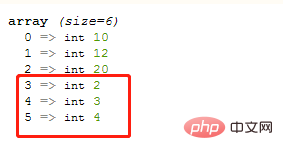1/1